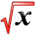# ProbabilityThis article is part of the EconHelp Tutoring WikiThis article is part of the MathHelp Tutoring Wiki

Probability theory is the branch of mathematics concerned with analysis of random phenomena.The central objects of probability theory are random variables, stochastic processes, and events: mathematical abstractions of non-deterministic events or measured quantities that may either be single occurrences or evolve over time in an apparently random fashion. Although an individual coin toss or the roll of a die is a random event, if repeated many times the sequence of random events exhibits certain statistical patterns, which can be studied and predicted.

As a mathematical foundation for statistics, probability theory is essential to many human activities that involve quantitative analysis of large sets of data. Methods of probability theory also apply to descriptions of complex systems given only partial knowledge of their state. In Economics, probability theory is a foundation for econometrics, which is the branch that involves testing economic theories and formulating models.

# Terminology

1 Sample: A sample is the part of population that is actually observed.

2 Sample Space: all possible outcomes from an experiment

3 Event: a subset of the sample space, to which a probability can be assigned

4 Conditional Probability: the probability of some event A, assuming event B

5 Collectively Exhaustive: N events are called collectively exhaustive when the union of these sets equals to the sample space.

6 Mutually Exclusive: Two events are called mutually exclusive if the intersection of them is empty.

# Probability Rules

{\begin{aligned}P(A\cup B)&=P(A)+P(B)-P(A\cap B)\\&=P(A)+P(B)\ if\ A\ and\ B\ are\ mutually\ exclusive\ \end{aligned}}Conditional Probability

$P(B|A)={\frac {P(A\cap B)}{P(A)}}$Statistical Independence

$P(A\cap B)=P(A)\cdot P(B)$Counting

$P_{x}^{n}={\frac {n!}{(n-x)!}}$$C_{x}^{n}={\frac {n!}{x!(n-x)!}}$# Bivariate Probabilities

Consider two sample spaces, each with H and K mutually exclusive and collectively exhaustive events respectively.

$P(A_{i})=\sum _{j=1}^{K}P(A_{i}\cap B_{j})\ for\ i=1,2,...,H$$P(B_{j})=\sum _{i=1}^{H}P(A_{i}\cap B_{j})\ for\ j=1,2,...,K$$\sum _{i=1}^{H}P(A_{i})=1\qquad \sum _{j=1}^{K}P(B_{j})=1$# Bayes'Theorem

Given two events A and B, Bayes' Theorem states the following:

$P(B|A)={\frac {P(A|B)P(B)}{P(A)}}$Suppose that $E_{1},E-2,...,E_{k}$are mutually exclusive and collectively exhaustive, we have $\sum _{j=1}^{k}P(E_{j})=1$.

Let A be some other event and consider conditional probabilities known for $P(A|E_{j})\ for\ j=1,2,...,k$, then

$P(E_{i}|A)={\frac {P(A|E_{i})P(E_{i})}{P(A)}}$$P(A)=\sum _{j=1}^{k}P(A|E_{j})P(E_{j})$